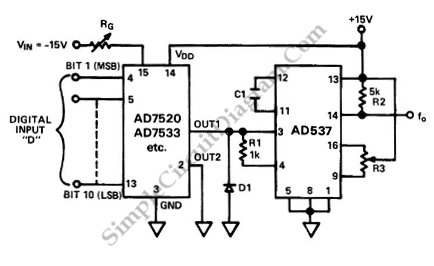# Digitally Programmable Oscillator

The schematic diagram below shows a digitally programmable oscillator circuit. This programmable oscillator circuit is based on V/F (voltage to frequency) converter, which is programmable by an analog voltage at the input. To make it digitally programmable, a DAC (digital to analog converter) is added to feed the V/F input. The components of the DAC is AD7520 multiplying D/A converter and  the component for the V/F converter is an AD537 monolithic converter. This circuit has excellent linearity in the range of 0 to 100 kHz. This circuit generates programmable square wave frequency. Here is the schematic diagram of the circuit: